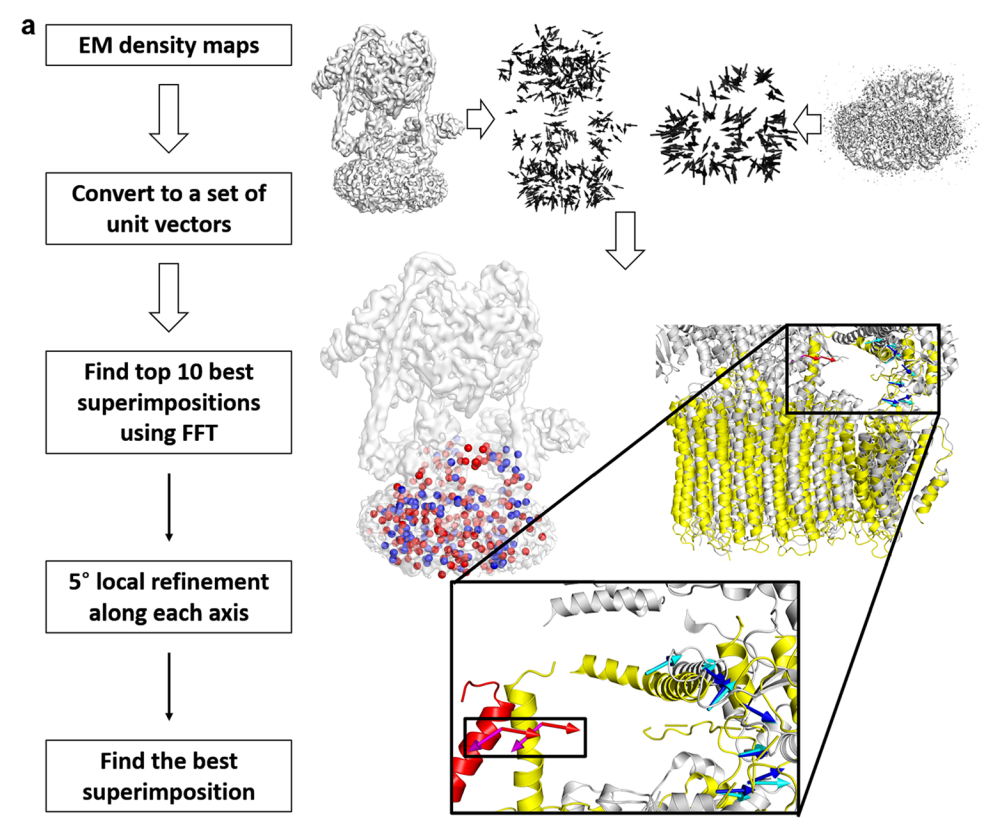## VESPER

VESPER uses a combination of mean shift algorithm and fast Fourier transform (FFT) to identify the best superimposition of two EM maps. Source codes are available here. Alignment using conventional Cross-correlation and the Laplacian filter is available in the provided code.

# VESPER Protocol

### Flowchart of VESPER# Availability

## Tech Specs

CPU: >=4 cores
Memory: >=32Gb
GPU: not required.

# Usage guide

## (1) Identify the best superimposition between two EM maps by VESPER.

```
Usage: VESPER -a [MAP1.mrc (large)] -b [MAP2.mrc (small)] [(option)]

---Options---
-t [float] : Threshold of density map1 def=0.000
-T [float] : Threshold of density map2 def=0.000
-g [float] : Bandwidth of the gaussian filter
def=16.0, sigma = 0.5*[float]
-s [float] : Sampling grid space def=7.0
-A [float] : Sampling Angle interval def=30.0
-c [int  ] : Number of cores for threads def=2
-N [int  ] : Refine Top [int] models def=10
-S: Show topN models in PDB format def=false
-V: Vector Products Mode def=true
-L: Overlap Mode def=false
-C: Cross Correlation Coefficient Mode def=false
Using normalized density value by Gaussian Filter
-P: Pearson Correlation Coefficient Mode def=false
Using normalized density value by Gaussian Filter and average density
-F: Laplacian Filtering Mode def=false
-E: Evaluation mode of the current position def=false
```

## (2) Calculate the normalized z-score for top 10 models from VESPER. Normalized z-scores for top 10 models are written into the output file.

```
python cluster_score.py:
usage: cluster_score.py [-h] -i INPUT_FILE [-c CUTOFF] [-o OUT_NAME]

optional arguments:
-h, --help            show this help message and exit
-i INPUT_FILE, --input INPUT_FILE
Required. Name of input file.
-c CUTOFF             Optional. Clustering cutoff ranging from 0 to 1.
Default = 0.2.
-o OUT_NAME, --output OUT_NAME
Optional. Name of output file. If not specified, the
output file would be named as input filename followed
by .normzscore.
```